Copyright © Michael Richmond. This work is licensed under a Creative Commons License.

# Thermodynamic Equilibrium, Local and otherwise

We are interested in the details of light's passage through the outer layers of a star. One of the crucial factors in this process is the "temperature" of the gas. But -- what exactly do we mean by "temperature"? Is it possible to define a temperature in these exotic regions?

It will turn out that one can define a "temperature" in some very strict sense only under special conditions, called thermodynamic equilibrium, which do not actually apply in the atmospheres of real stars. However, for many purposes, one can define a sort of local thermodynamic equilibrium which is good enough to bring theory and observation into agreement.

#### The many different temperatures

Let's count the ways we could try to describe the temperature of the photosphere of a star.

Effective Temperature
Measure the radius and luminosity of a star. Find the temperature of a blackbody which would produce the same total amount of energy:Color Temperature
Measure the spectrum of a star, or, more commonly, its color via the difference of magnitudes in two filters.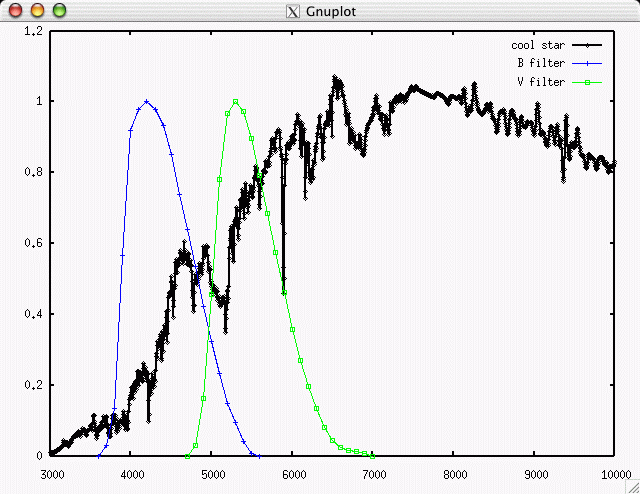Find the temperature of a blackbody which would produce a spectrum of the same shape, or the same difference in magnitudes when observed through those filters.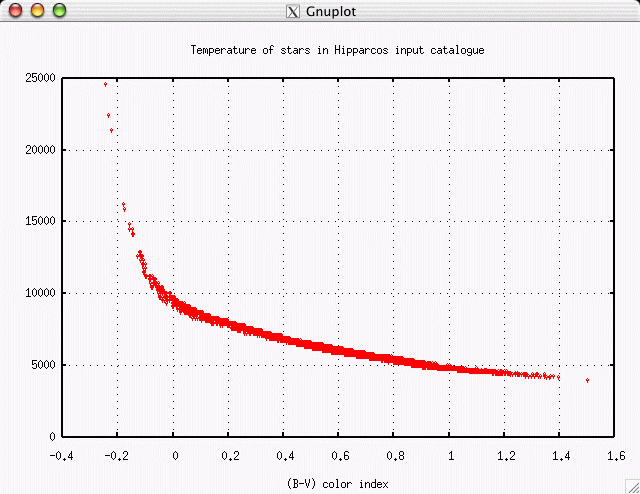Excitation temperature
Measure the ratio of atoms in two energy levels of some neutral atom; say, the n=2 to n=1 ratio of hydrogen. Using the Boltzmann equation,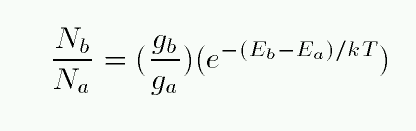determine the temperature which would reproduce the observed ratio.

Ionization temperature
Measure the ratio of atoms in two ionization stages; say, ionized hydrogen to neutral hydrogen. Using the Saha equation,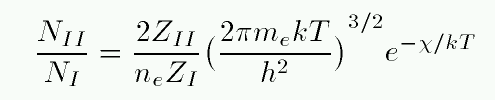determine the temperature which would reproduce the observed ratio.

Kinetic temperature
Measure the speed of particles in the gas. If they follow a Maxwell-Boltzmann distribution,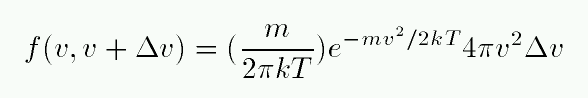determine the temperature which would reproduce the observed distribution. This is often simplified to "measure some average speed of particles, and compare to the average speed of a Maxwell-Boltzmann distribution."

Exercise:
1. Which of these is simplest to measure, in practice? What observations would you need?
2. Which of these is hardest to measure, in practice? What observations would you need?

In some very simple situations, all these temperatures might be exactly the same. For example, if we build a box, fill it with some gas, heat the walls of the box to a uniform and steady temperature, and wait a bit, we will find that a single temperature T will fit into all of these equations. In such an ideal case, we say that the gas has achieved thermodynamic equilibrium.

Two of the hallmarks of thermodynamic equilibrium are isotropy -- properties are the same in all directions -- and homogeneity -- properties are the same in all places. If we consider an entire star as a whole, neither condition is true: the center of a star is much hotter than its outer regions, for example.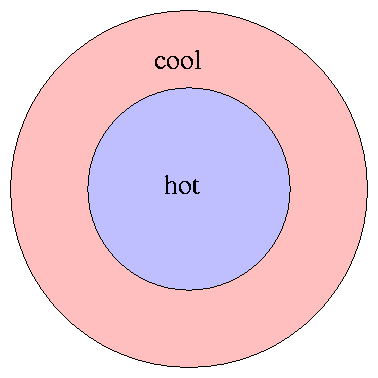Rats! If we are trying to understand the detailed interaction of light and matter inside a star, it would be very, very convenient if we could use a single value, T, to predict the speed of gas molecules, the excitation of atoms, the spectrum of radiation, etc. But it appears that real life is more complicated ....

... unless, perhaps, we can find some way to limit ourselves to a small enough piece of the star that matter and radiation are at least approximately in thermodynamic equilibrium.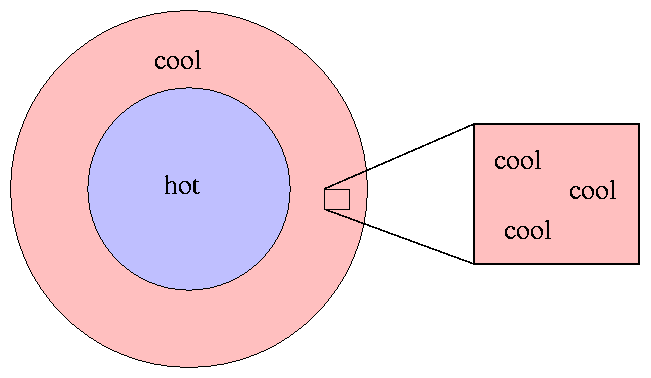But how small does the piece have to be?

#### Mean free path (mfp)

It will soon become important to know how far an atom, molecule, electron, photon -- let's just say some "particle" -- will travel through the tenuous gas of the atmosphere before running into something.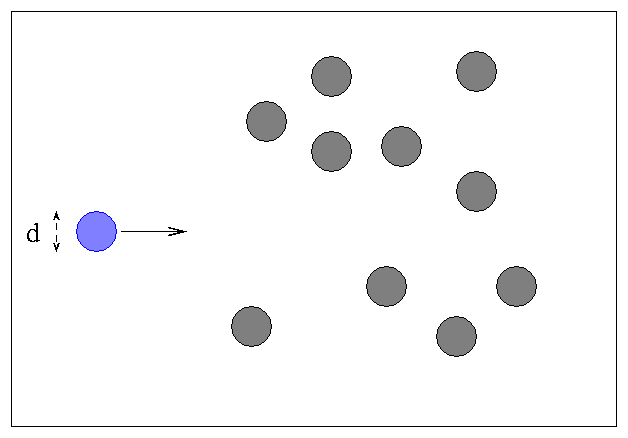If we have a gas of atoms with typical diameter d and radius r = d/2, with density n atoms per unit volume, the question is -- how far will the blue particle typically travel before it strikes an atom? We can simplify the calculations by imagining an equivalent situation, in which the blue particle becomes twice as big and all the other particles shrink down to perfect little points: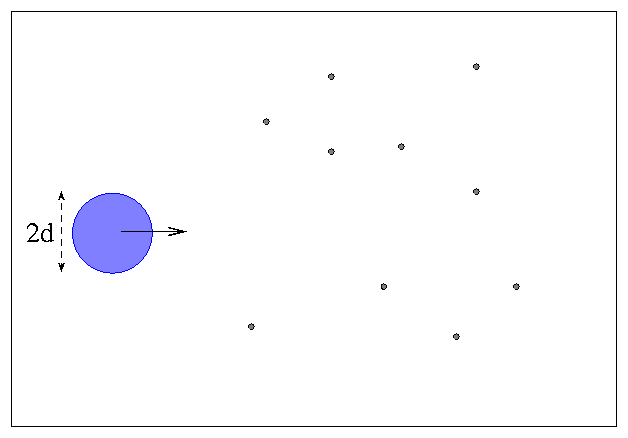Collisions between the blue particle and others will occur just as frequently now as in real life, but we don't have to worry about the mathematics of intersecting circles anymore.

Now, as the blue particle moves through the sea of other particles, it will sweep out a cylindrical path ...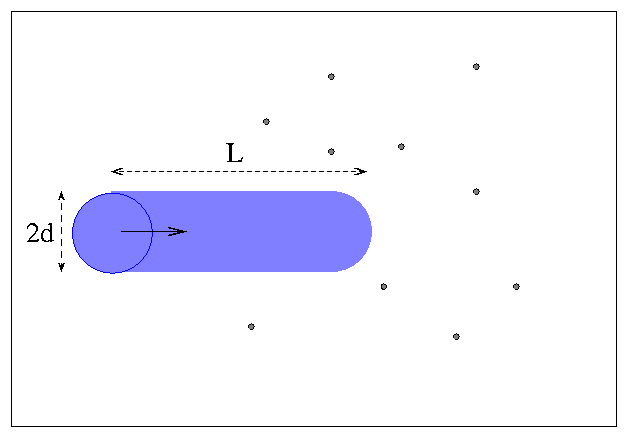The mean free path is the average distance the blue particle must move until another particle falls within the volume it sweeps out. That's just another way of describing the distance between collisions.

Exercise:
1. Express the volume of the swept-out volume as a function of the distance L.
2. At what distance will an average of one particle fall within the volume?
3. Here in this classroom, the density of oxygen molecules is about 1.0 x 10^(-3) g/cc. Estimate the mean free path of an oxygen molecule.
4. In the solar photosphere, the density of hydrogen atoms is about 2.5 x 10^(-7) g/cc. Estimate the mean free path of a hydrogen atom.

#### Local Thermodynamic Equilibrium

Consider the motion of an individual atom in the stellar photosphere. We know that the temperature must gradually decrease from the center outwards towards the edge. If the mean free path (mfp) of the atom typically takes it from a region of one temperature to a region with a different temperature,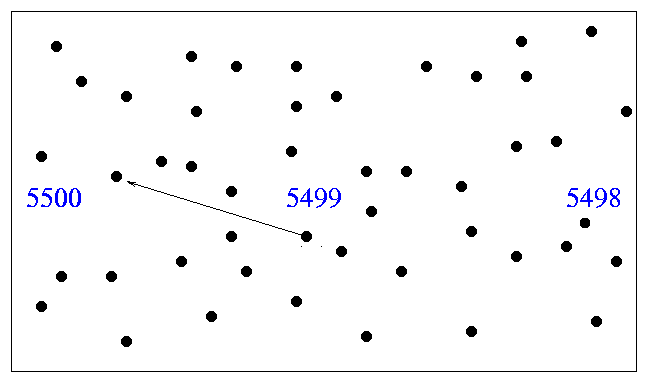then it will constantly be changing its properties with every collision. That means that we will never be able to describe it for any length of time as having some well defined temperature.

On the other hand, if the mean free path of the atom is very small compared to the distance over which the temperature changes,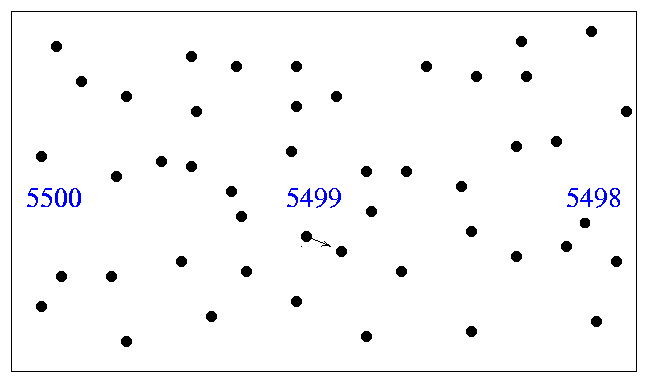then the atom will collide many times with other atoms, all of the same temperature, before it can possibly reach some region with a different temperature. In this case, the speed of atoms within some small region may very well be described by a Maxwell-Boltzmann distribution with a definite temperature.

So, over what distance does the temperature change in the sun's photosphere? It sounds like a simple question, but what does it mean?

• over what distance does the temperature change by 100 degrees?
• over what distance does the temperature change by 1000 degrees?
• over what distance does the temperature change by a factor of 2?
• over what distance does the temperature change by a factor of e?

Physicists often choose to define the distance over which some property changes "significantly" as the ratio of the LOCAL value to the LOCAL GRADIENT:The distance defined in this way is called the (temperature) scale height.

Use the graph below to estimate the temperature scale height of the solar photosphere: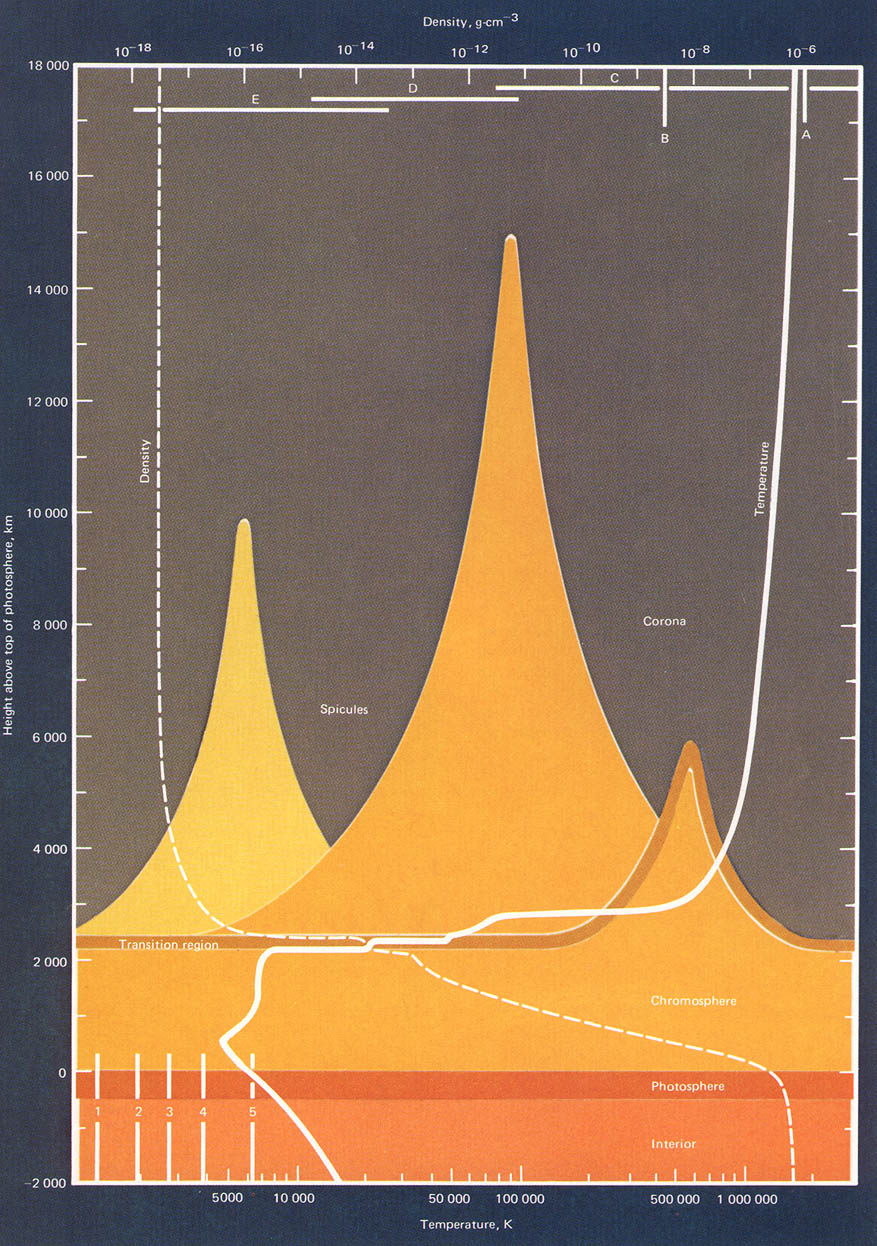Exercise:
1. What is the temperature in the middle of the photosphere?
2. How much does the temperature change from top to bottom of photosphere?
3. What is the distance from top to bottom of photosphere?
4. Calculate the scale height H of the solar photosphere.

Compare the scale height H of the solar photosphere to the mean free path of its hydrogen atoms. May we consider the atoms in the photosphere to be in LTE?Copyright © Michael Richmond. This work is licensed under a Creative Commons License.